## Monday, August 22, 2011

### SVAR Estimation

For the next phase of my GSoC project, I integrated SVAR estimation with restrictions on the within period effects and shock identification. This follows the method outlined in section 11.6 of Hamilton (1994) . In order to show how the system works, I will work through an example.

Structural VARs of the type I will be positing here are best suited to situations where impulse response function shocks need to be identified, but there is little to no theoretical justification for the within period dynamics required for orthogonalization. While this allows greater flexibility to the researcher in specifying the model, it also leaves greater room for error, especially when specifying what parameters can be assumed zero and what should be estimated, and whether or not this specification meets the criteria for shock identification.

Given time series data, SVAR class, initiation requires (at a minimum):

1) svar_type
2) A, B matrices marking with an 'E' or 'e' where parameters are unknown

This must be A,B, or AB. An 'A' system assumed that the matrix premulitplying the error matrix is the identity matrix and vice-versa for the 'B' system. In an 'AB' system, elements in both the A and B matrix need to be estimated. The system can be summarized as: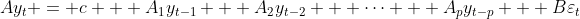We estimate A, B in a second stage after A_1-A_n, /Sigma_u are estimated via OLS, by maximizing the log-likelihood: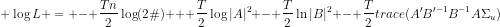We could set up an example system as:

In : import numpy as np

In : A = np.array([[1, 'E', 0], [1, 'E', 'E'], [0, 0, 1]])

In : A
Out:
array([['1', 'E', '0'],
['1', 'E', 'E'],
['0', '0', '1']],
dtype='|S1')

In : B = np.array([['E', 0, 0], [0, 'E', 0], [0, 0, 'E']])

In : B
Out:
array([['E', '0', '0'],
['0', 'E', '0'],
['0', '0', 'E']],
dtype='|S1')



In order to aid numerical maximum likelihood estimation, the SVAR class fit can also be passed guess matrices for both A and B parameters.

Building upon the previously used three variable macro system, a simple example of an AB system estimation is as follows:

import numpy as np
import scikits.statsmodels.api as sm
from scikits.statsmodels.tsa.api import VAR, SVAR
import matplotlib.pyplot as plt

mdata = mdata[['realgdp','realcons','realinv']]
names = mdata.dtype.names
data = mdata.view((float,3))
data = np.diff(np.log(data), axis=0)

#define structural inputs
A = np.asarray([[1, 0, 0],['E', 1, 0],['E', 'E', 1]])
B = np.asarray([['E', 0, 0], [0, 'E', 0], [0, 0, 'E']])
A_guess = np.asarray([0.5, 0.25, -0.38])
B_guess = np.asarray([0.5, 0.1, 0.05])
mymodel = SVAR(data, svar_type='AB', A=A, B=B, names=names)
res = mymodel.fit(maxlags=3, maxiter=10000, maxfun=10000, solver='bfgs')
res.irf(periods=30).plot(impulse='realgdp', plot_stderr=False)



From here, we can access the estimates of both A and B:

In : res.A
Out:
array([[ 1.        ,  0.        ,  0.        ],
[-0.50680204,  1.        ,  0.        ],
[-5.53605672,  3.04117688,  1.        ]])

In : res.B
Out:
array([[ 0.00757566,  0.        ,  0.        ],
[ 0.        ,  0.00512051,  0.        ],
[ 0.        ,  0.        ,  0.02070894]])



This also produces a SVAR irf, resulting from a impulse shock to realgdp:

We can check our work by comparing our work to comparable packages (see below) and also performing some simple calculations. If we calculated A and B correctly, then: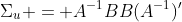We find:

In : P = np.dot(npl.inv(res.A), res.B)

In : np.dot(P,P.T)
Out:
array([[  5.73906806e-05,   2.90857141e-05,   2.29263262e-04],
[  2.90857141e-05,   4.09603703e-05,   3.64524316e-05],
[  2.29263262e-04,   3.64524316e-05,   1.58721639e-03]])

In : res.sigma_u
Out:
array([[  5.73907395e-05,   2.90857557e-05,   2.29263451e-04],
[  2.90857557e-05,   4.09604397e-05,   3.64524456e-05],
[  2.29263451e-04,   3.64524456e-05,   1.58721761e-03]])



Through much trial, it seems like the 'bfgs' estimator is the best for solving the maximum likelihood problem. I would like to investigate in the future why this may be. Estimating the likelihood was aided greatly by bringing in the likelihood methods already present in the base component of the statsmodels package.

This equivalent system can be estimated and plotted in the R  script:

library("vars")

names <- colnames(data)
data <- log(data[c('realgdp','realcons','realinv')])
data <- sapply(data, diff)
data = ts(data, start=c(1959,2), frequency=4)

amat <- matrix(0,3,3)
amat[1,1] <- 1
amat[2,1] <- NA
amat[3,1] <- NA
amat[2,2] <- 1
amat[3,2] <- NA
amat[3,3] <- 1
bmat <- diag(3)
diag(bmat) <- NA
svar <- SVAR(var, estmethod = 'scoring', Bmat=bmat, Amat=amat)
#myirf <- plot(irf(myvar, impulse = "realgdp", response = c("realgdp", "realcons", "realinv"), boot=TRUE, n.ahead=30, ci=0.95))
#plot.irf()



This is the end of my Google Summer of Code project. In the future, I hope to continue work on SVAR, bring in long-run restrictions ala Blanchard and Quah, and further test solvers and their performance. I have benefited a lot from this project and would like to sincerely thank my mentors: Skipper Seabold, Josef Perktold, and Alan Isaac. They have been a great support and have answered my questions swiftly and completely. All blame for failure to complete the goals I set for myself at the beginning of the summer rests on me alone. Not only have I learned a lot about time series econometrics, but also quite a bit about how community software development works and especially realistic timelines. This has been an invaluable experience and I plan to further improve my contributions to the project in the coming year.

Bart Baker

 Hamilton, James. 1994. Time Series Analysis. Princeton University Press: Princeton.
 R Development Core Team. 2011. R: A Language and Environment for Statistical Computing. R FOundation for Statistical Copmuting.

## Tuesday, July 19, 2011

### Sims and Zha IRF Error Bands

After completing the Monte Carlo error bands, I moved onto integrating Sims and Zha error bands into the statsmodels package.These are based on pages 1127 to 1129 of Chris Sims and Tao Zha's 1999 Econometrica (Vol. 67 No. 5) article, "Error Bands for Impulse Responses."

This method took a long time just to get my head around and a lot of trial and error. While Sims and Zha focus on Bayesian sampling methods from the joint distribution of the coefficients and covariance matrix to generate the draws of the MA(n) representations, the method used here to generate these draws are simpler Monte Carlo simulations. In order for these error bands to truly follow the prescription of Sims and Zha (SZ), the Bayesian sampling methods would need to be employed.

Here's a quick overview of the theory.

Given a covariance matrix Sigma, we can perform eigenvalue decomposition as such: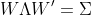where Lambda is diagonal and each diagonal element of Lambda corresponds to an eigenvalue of Sigma. Column 'k' of W is the eigenvector corresponding to the 'k'th diagonal element of Lambda or the 'k'th eigenvector.

We will also define our moving average representation or impulse response function as: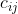where c is the time series vector ('t' to 't+h') response of variable 'i' to a shock in period 't' to variable 'j'.

SZ make the case when the time series model (VAR) is fit to data that is not smooth (differenced, etc.), most of the variation will be contained in the principal components of W. With this information, SZ propose three methods for characterizing the uncertainty around the data.

The following three arrays of graphs can be produced by the following code:

import numpy as np
import scikits.statsmodels.api as sm
from scikits.statsmodels.tsa.api import VAR
import matplotlib.pyplot as plt

mdata = mdata[['realgdp','realcons','realinv']]
names = mdata.dtype.names
data = mdata.view((float,3))
data = np.diff(np.log(data), axis=0)
mymodel = VAR(data,names=names)
res = mymodel.fit(maxlags=3,ic=None)

res.irf(periods=20).plot(impulse='realgdp', stderr_type='sz1', repl=1000, seed=30)
res.irf(periods=20).plot(impulse='realgdp', stderr_type='sz2',repl=1000, seed=30)
res.irf(periods=30).plot(impulse='realgdp', stderr_type='sz3', repl=1000, seed=30)
plt.show()



At this point, I have only implemented this for the non-orthogalized impulse responses, but Sims and Zha explicitly address this case in their paper and it is analogous to the methods defined below.

1) Symmetric, assumes Gaussian uncertainty. These error bands add and subtract the estimated impulse response functions with error completely defined by the principal component1: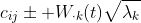where W_{.k} is the column of W corresponding to the 'k'th eigenvalue of Sigma. The above equation would be the 68% probability bands. 95% would simply be mulitplied by a scalar of 1.96 on both sides.

In our three variable model, this method produces the following error bands a response to a gdp shock:

Looks nice enough. These are defined as implemented to be symmetric, so I wasn't too worried about these.

2) Non-symmetric error bands generated by Monte Carlo draws where only covariance between time but not across variables exists.

In this case, instead of assuming Gaussian uncertainty, we retain the draws used to estimate the initial covariance matrix, Sigma, and for each of the these draws we calculate the vector gamma_k: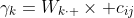where W_{k.} is the 'k'th row of W, and k refers to the largest eigenvalue of Sigma. Using these quantiles of the individual elements of gamma_k across the MC draws, we can generate 68% probability bands as follows: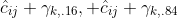where the subscripts on gamma_k refer to the 16th and 84th percentile of the gamma draws. In our three variable case, this produces the following graphical representation:

We can see that in this case the uncertainty clearly drops precipitously once we hit a certain t in the time series representation.

Just for completeness, if we look at the response of the same variables to a shock to real consupmtion, we notice that this method does not even guarantee that the probability bands contain the estimated impulse response function:

While this was extremely worrisome at first, Sims and Zha do give some examples where the probability bands do not contain the estimated impulse response function for certain 't'. These error bands are supposed to give the researcher an idea as to the symmetry (or lackthereof) of the posterior distribution of the impulse response functions.

3) Non-symmetric error bands generated by Monte Carlo draws where covariance between time and between variables is identified.

Here, instead of treating each vector of responses individually, we consider each set of impulse response functions as a single system. Sims and Zha note that while in most cases the majority of the covariance will be between intertemporal observations of a single variable, considering inter-variable time series covariance may be valuable in certain situations.

In order to investigate how different c_ij related to each other, we stack each set of impulse response functions that respond to a single shock j and then compute the eigenvector decomposition of this stacked vector for each MC draw. This allows us to compute eigenvectors that contain the variation both across time periods and across variables. We calculate our gamma_k in an analagous manner to the second method and add the appropriate gamma_k quantiles to the estimated response.

It happens that in our system, the cross-variable covariance does not reveal much addition information about a shock to GDP:

It seems as though the variation in real GDP dominates the variation in the other variables.

This can also be seen by examining the response of real GDP to all three shocks:

Altogether these methods are meant to be used to examine the characteristics of the time series representation of the data. The first Sims and Zha method would most likely be the one to publish in a paper, but the other two methods give the researcher a more complete picture of the nature of the posterior distribution.

A few small things still need to be worked out for the Sims and Zha methods to be a complete part of the VAR package. As I mentioned before, they still need to be implemented for orthogonalized IRFs, but this will not be difficult (they are very clear in their paper in moving towards this implementation). Also, it will be important to bring in user choice when it comes to which principal component to use when characterizing the data. For Sims and Zha error bands 1 and 2, the user can pass in a matrix of integers that correspond to the chosen principal components of the variance-covariance matrix.

Scheduling here on out (let me know what you think, updated):

1) I'd like to completely integrate Sims and Zha error bands. Specifically, this means:

a) Component choice for SZ3

b) Orthogonalized error bands

c) Clean up code

I will aim to finish the above tasks by this Saturday (7/23).

2) After this, I would like to move on to structural VAR implementation. This in itself will feed back on the error band methods that I have been working on. SVAR will draw from the ML methods already present in statsmodels. I'd like to finish a SVAR estimation method by the August 3rd.

3) Once SVAR is completely integrated into the package, per Skipper's suggestion, I will be using pure Python to generalize the Kalman filter. I'll have more questions once I reach that point.

I'd really like to move much quicker than I did in the first half of GSOC and hit these goals.

Bart

1. SZ suggest using the largest eigenvalue(s) of Lambda, as they will most likely identify the majority of the variation in this type of data.

## Saturday, June 11, 2011

### Monte Carlo Standard Errors for Impulse Response Functions

I've come to a major checkpoint in integrating Monte Carlo error bands
for impulse response functions (this is only non-orthogonal right now).

Here is some quick code to get VAR IRF MC standard errors:

import numpy as np
import scikits.statsmodels.api as sm
from scikits.statsmodels.tsa.api import VAR
import matplotlib.pyplot as plt

mdata = mdata[['realgdp','realcons','realinv']]
#mdata = mdata[['realgdp','realcons','realinv', 'pop', 'realdpi', 'tbilrate']]
names = mdata.dtype.names
data = mdata.view((float,3))
data = np.diff(np.log(data), axis=0)
mymodel = VAR(data,names=names)
res = mymodel.fit(maxlags=3,ic=None)

#first generate asymptotic standard errors (to compare)
res.irf(periods=30).plot(orth=False, stderr_type='asym')
#then generate monte carlo standard errors
res.irf(periods=30).plot(orth=False, stderr_type='mc', seed=29, repl=1000)
plt.show()



Produces the following plots of a shock to (delta) realgdp.

Asymptotic:

Monte Carlo:

some of the pre-existing functions in these classes and also to
plotting.py. Because Monte Carlo standard errors are in general not
symmetric, I had to alter the plot_with_error function from the
tsa.vector_ar.plotting.py file and number of other functions.

VARResults.stderr_MC_irf(self, orth=False, repl=1000, T=25,
signif=0.05, seed=None)
This function generates a tuple that holds the lower and upper error
bands generated by Monte Carlo simulations.

IRAnalysis.cov_mc(self, orth=False, repl=1000, signif=0.05, seed=None)
This just calls the stderr_MC_irf function from the original model
using the number of periods specified when the irf() class is called
from the VAR class.

Modified functions:

BaseIRAnalysis.plot
Added specification of error type stderr_type='asym' or 'mc' (see
example). Also repl (replications) and seed options added if 'mc'
errors are specified.

in tsa.vector_ar.plotting.py
plot_with_error()
irf_grid_plot()

These functions now take the error type specified in the plot()
function above and treats the errors accordingly. Because of how all
of the VAR work (esp. plotting) assumed asymptotic standard errors,
all of these irf plot functions assumed that errors were passed in as
a single matrix with each standard error depending on the MA lag
length and shock variable. Now, depending on which error is specified,
the function will take a tuple of arrays as the standard error if
(stderr_type='mc') rather than a single array.

A serious issue right now is speed. While the asymptotic standard
errors take about a half second on my home laptop to run, the Monte
Carlo standard errors with 3 variables and 1000 replications takes
observations. The most taxing aspect of generating the errors is
re-simulating the data assuming normally distributed standard errors
1000 times (using the util.varsim() function).

Bart

## Monday, May 23, 2011

### 5/6 - 5/20: Getting Acclimated and VARRresults.reorder() function

Just finished up the official first two weeks of GSoC. I spent most of the first week attempting to get a feel for how all of the time series methods for statsmodels are organized. Once I felt comfortable within the VAR package, I began work on adding a reorder() function to the VARResults class, which allows the user to specify the order of the endogenous variables in a vector auto-regression system. While the order of the variables plays no role in estimating the system, if the shocks are to be identified, variable order is used to specify the within period impact of shocks to individual variables in the system.

For example, let us say that we have a 3-variable VAR system, originally ordered realGDP, realcons, and realinv, contained within the VARResults class 'res'. Reordering the variables in the system is as simple as follows:

In : import scikits.statsmodels.api as sm

In : import numpy as np

In : mdata = mdata[['realgdp','realcons','realinv']]

In : names = mdata.dtype.names

In : data  = mdata.view((float,3))

In : from scikits.statsmodels.tsa.api import VAR

In : res = VAR(data, names=names).fit(maxlags=3,ic=None)

In : res.names
Out: ['realgdp', 'realcons', 'realinv']

In : res_re = res.reorder(['realinv','realcons','realgdp'])

In : res_re.names
Out: ['realinv', 'realcons', 'realgdp']



The reorder function reuses all of the results from the original VAR class, but rearranges them to be in line with the new system. If working with large a # of observations, the computational advantage becomes useful pretty quickly. For example, with a 100,000 observation system with three variables, re-estimating the system after changing the variable order took 3.37 seconds, while using the reorder function took 0.57 seconds.

In the next few weeks I am planning on adding more impulse response function error band estimation methods. The current package only includes analytical error bands.

## Saturday, April 23, 2011

### Statsmodels: GSoC Prelim

This is my first entry in my Statsmodels Project Summer 2011 blog. I will update this blog weekly as the summer goes by with information regarding progress on my work for the scikits.statsmodels Python library.

In order to complete the preparation process for the statsmodels Google Summer of Code sponsorship, I wrote a quick patch that included a cointegration test. As of now, the test can only be run on a bivariate system with a simple Dickey-Fuller test on the residuals, using the Mackinnon  critical values. I would like to expand the functionality of this test to allow for Augmented Dickey-Fuller tests of the residuals and also tests of multivariate cointegrated systems. This patch served as a nice way to dive into what time series methods scikits.statsmodels currently includes in its toolbox and where to go from here.

## Tuesday, June 22, 2010

### Statsmodels: GSoC Week 4 Update

I spent the last week finishing up the paper that I submitted to accompany my talk at the SciPy conference. I am really looking forward to going to Austin and hearing all the great talks (plus I hear the beer is cheap and the food and music are good, which doesn't hurt). In addition to finishing up the paper, I have started to clean up our time series code.

So far this has included finishing the augmented Dickey-Fuller (ADF) test for unit roots. The big time sink here is that the ADF test-statistic has a non-standard distribution in most cases.  The ADF test statistic is obtained by running the following regression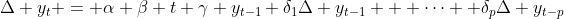One approach to testing for a unit root means testing the t-stat on the coefficient on the lagged level of y.  The actual distribution for this statistic, however, is not Student's t.  Many software packages use the tables in Fuller (1976, updated to 1996 version or not) in order to get the critical values for the test statistic depending on the sample size.  They use linear interpolation for sample sizes not included in the table.  The p-values for the obtained test statistic are usually obtained using MacKinnon's (1994) study that estimated regression surfaces of these distributions via Monte Carlo simulation.

While we do use MacKinnon's approximate p-values from the 1994 paper, MacKinnon wrote a note updating this paper in early 2010, which gives new regression surface results for obtaining the critical values.  We use these new results for the critical values.  Therefore, when using our ADF test, it is advised that if the p-value is close to the reject/accept region then the critical values should be used in place of the p-value to make the ultimate decision.

We can illlustrate the use of ADF.  Note that this version is only in my branch and that it is still in the sandbox, even though it has now been tested, because the API and returned results may change.  We will demonstrate on a series that we can easily guess is non-stationary, real GDP.

In : import scikits.statsmodels as sm

In : from scikits.statsmodels.sandbox.tsa.stattools import adfuller

In : realgdp = data.data['realgdp']

Out:
(-1.8566384063254346,
0.67682917510440099,
4,
198,
{'1%': -4.0052351400496136,
'10%': -3.1402115863254525,
'5%': -3.4329000694218998})


The return values are the test statistic, its p-value (the null-hypothesis here is that the series does contain a unit root), the number of lags of the differences used, the number of observations for the regression, and a dictionary containing the critical values at the respective confidence levels.  The regression option controls the type of regression (ie., whether to include a constant or a linear or quadratic time trend), and the autolag option has three options for choosing the lag length to help correct for serial correlation in the regression.  There are 'AIC', 'BIC', and 't-stat'.  The former two choose the lag length that maximizes the infofrmation criterion, the latter chooses the lag length based on the significance of the lag.  This starts with maxlag and works its way down.  The docstring has more detailed information.

Beyond this, I have been working on an autocorrelation function (acf), a  partial autocorrelation function (pacf), and Q-Statistics (Box-Ljung test). Next up for this week is finishing my VAR class with identification schemes.  After this, I will work to integrate post-estimation tests into our results classes, most likely using some sort of mix-in classes and attach test containers to the results objects for test results.  Then it's off to the SciPy conference. There I will hopefully be participating in the stats sprint, helping out with the docs marathon and discussing what we need for the future of statistics and Python.

Fuller, W.A.  1996.  Introduction to Statistical Time Series. 2nd ed.  Wiley.

MacKinnon, J.G. 1994.  "Approximate asymptotic distribution functions for
unit-root and cointegration tests.  Journal of Business and Economic
Statistics 12, 167-76.

MacKinnon, J.G. 2010. "Critical Values for Cointegration Tests."
Queen's University, Dept of Economics, Working Papers.  Available at
http://ideas.repec.org/p/qed/wpaper/1227.html


## Friday, June 11, 2010

### Statsmodels: GSoC Week 3 Update

[Edit: Formatting should be fixed now. I will not be reformatting old posts though, so that they don't get reposted at Planet SciPy]

Last week was spent mainly ensuring that I pass my comps and remain a PhD student. This week was much more productive for coding. For now, all changes are in my branch and have not been merged to trunk, but I will describe the two big changes.

The first concerns the datasets package. This one is not all that exciting, but suffice it to say that the datasets are now streamlined and use the Bunch pattern to load the data. Thanks, Gaël, for pointing this out. I also rewrote a bit of David's datasets proposal from scikits-learn to reflect the current design of our datasets and thoughts. You can see it here (soon to be on the docs page). We are making an effort to ensure that our datasets are going to be similar to those of scikits-learn.

The second change was an improvement of the fitting of maximum likelihood models and the start of a GenericLikelihoodModel class. Maximum likelihood based models (mainly discrete choice models in the main code base right now) can now be fit using any of the unconstrained solvers from scipy.optimize (Nelder-Mead, BFGS, CG, Newton-CG, Powell) plus Newton-Raphson. To take a simple example to see how it works, we can fit a Probit model.

In : import scikits.statsmodels as sm

In : res_newton = sm.Probit(data.endog, data.exog).fit(method="newton")
Optimization terminated successfully.
Current function value: 12.818804
Iterations 6

In : res_nm = sm.Probit(data.endog, data.exog).fit(method="nm", maxiter=500)
Optimization terminated successfully.
Current function value: 12.818804
Iterations: 439
Function evaluations: 735

In : res_bfgs = sm.Probit(data.endog, data.exog).fit(method="bfgs")
Optimization terminated successfully.
Current function value: 12.818804
Iterations: 15
Function evaluations: 21

In : res_cg = sm.Probit(data.endog, data.exog).fit(method="cg", maxiter=250)
Optimization terminated successfully.
Current function value: 12.818804
Iterations: 188
Function evaluations: 428

In : res_ncg = sm.Probit(data.endog, data.exog).fit(method="ncg", avextol=1e-8)
Optimization terminated successfully.
Current function value: 12.818804
Iterations: 12
Function evaluations: 14
Hessian evaluations: 12

In : res_powell = sm.Probit(data.endog, data.exog).fit(method="powell", ftol=1e-8)
Optimization terminated successfully.
Current function value: 12.818804
Iterations: 12
Function evaluations: 568

All of the options for the solvers are available and are documented in the fit method. As you can see, some of the default values need to be changed to ensure (accurate) convergence. The Results objects that are returned have two new attributes.

In : res_powell.mle_retvals
Out:
{'converged': True,
'direc': array([[ 7.06629660e-02, -3.07499922e-03, 5.38418734e-01,
-4.19910465e-01],
[ 0.00000000e+00, 1.00000000e+00, 0.00000000e+00,
0.00000000e+00],
[ 1.49194876e+00, -6.64992809e-02, -6.96792443e-03,
-3.22306873e+00],
[ -5.36227277e-02, 1.18544093e-01, -8.75205765e-02,
-2.42149981e+00]]),
'fcalls': 568,
'fopt': 12.818804069990534,
'iterations': 12,
'warnflag': 0}

In : res_powell.mle_settings
Out:
{'callback': None,
'disp': 1,
'fargs': (),
'ftol': 1e-08,
'full_output': 1,
'maxfun': None,
'maxiter': 35,
'optimizer': 'powell',
'retall': 0,
'start_direc': None,
'start_params': [0, 0, 0, 0],
'xtol': 0.0001}

The dict mle_retvals contains all of the values that are returned from the solver if the full_output keyword is True. The dict mle_settings contains all of the arguments passed to the solver, including the defaults so that these can be checked after the fit. Again, all settings and returned values are documented in the fit method and in the results class, respectively.

Lastly, I started a GenericLikelihoodModel class. This is currently unfinished, though the basic idea is laid out. Take again the Probit example above using Lee Spector's educational program data. And assume we didn't have the Probit model from statsmodels. We could use the new GenericLikelihoodModel class. There are two ways (probably more) to proceed. For those comfortable with object oriented programming and inheritance in Python, we could subclass the GenericLikelihoodModel, defining our log-likelihood method.

from scikits.statsmodels import GenericLikelihoodModel as LLM
from scipy import stats
import numpy as np

class MyModel(LLM):
def loglike(self, params):
"""
Probit log-likelihood
"""
q = 2*self.endog - 1
X = self.exog

Now this model could be fit, using any of the methods that only require an objective function, i.e., Nelder-Mead or Powell.

In : mod = MyModel(data.endog, data.exog)

In : res = mod.fit(method="nm", maxiter=500)
Optimization terminated successfully.
Current function value: 12.818804
Iterations: 439
Function evaluations: 735

In : res_nm.params
Out: array([ 1.62580058, 0.05172931, 1.42632242, -7.45229725])

In : res.params
Out: array([ 1.62580058, 0.05172931, 1.42632242, -7.45229725])

The main drawback right now is that all statistics that rely on the covariance of the parameters, etc. will use numeric gradients and Hessians, which can lessen that accuracy of those statistics. This can be overcome by providing score and hessian methods as loglike was provided above. Of course, for more complicated likelihood functions this can soon become cumbersome. We are working towards more accurate numerical differentiation and discussing options for automatic or symbolic differentiation.

The main advantage as opposed to just writing your likelihood and passing it to a solver is that you have all of the (growing number of) statistics and tests available to statsmodels right in the generic model.

I would also like to accommodate those who are less familiar with OOP and inheritance in Python. I haven't quite worked out the final design for how this would go yet. Right now, you could do the following, though I don't think it quite meets the less complicated goal.

In : from scikits.statsmodels.model import GenericLikelihoodModel as LLM

In : import scikits.statsmodels as sm

In : from scipy import stats

In : import numpy as np

In :

In :

In : def probitloglike(params, endog, exog):
....: """
....: Log likelihood for the probit
....: """
....: q = 2*endog - 1
....: X = exog
....:

In : mod = LLM(data.endog, data.exog, loglike=probitloglike)

In : res = mod.fit(method="nm", fargs=(data.endog,data.exog), maxiter=500)
Optimization terminated successfully.
Current function value: 12.818804
Iterations: 439
Function evaluations: 735

In : res.params
Out: array([ 1.62580058, 0.05172931, 1.42632242, -7.45229725])

There are still a few design issues and bugs that need to be worked out with the last example, but the basic idea is there. That's all for now.

## Monday, May 31, 2010

### Week 1 GSoC Update

Last week was the first of the Google Summer of Code. I spent most of the week in a Bayesian econometrics class led by John Geweke and studying for a comprehensive exam that I take this week, so progress on statsmodels was rather slow. That said, I have been able to take care of some low hanging fruit.

There are a few name changes:

statsmodels/family -> statsmodels/families
statsmodels/lib/io.py -> statsmodels/iolib/foreign.py

Also Vincent has done a good bit of work on improving our output using the SimpleTable class from econpy. I will post some examples over the coming weeks, but SimpleTable provides an easy way to make tables in ASCII text, HTML, or LaTeX. The SimpleTable class has been moved

statsmodels/sandbox/ouput.py -> statsmodels/iolib/table.py

Beyond the renames, I have removed the soft dependency on RPy for running our tests in favor of hard-coded results, refactored our tests, and added a few additional ones along the way.

We are also making an effort to keep our online documentation synced with the current trunk. The biggest change to our documentation is the addition of a developer's page for those who might like to get involved. As always, please report problems with the docs on either the scipy-user list or join in the discussions of statsmodels, pandas, larry, and other topics on statistics and Python at the pystatsmodels Google group.

## Saturday, May 1, 2010

### Plans for the Summer

A quick update on the plans for statsmodels over the next few months.

I have been accepted for my second Google Summer of Code, which means that we will have a chance to make a big push to get a lot of our work out of the sandbox, tested, and included in the main code base.

The quick version follows. As far as general issues, I will be getting the code ready for Python 3 and focusing on some design issues including an improved generic maximum likelihood framework, post-estimation testing, variable name handling, and output in text tables, LaTeX, and html. I will then be working to get a lot of our code out of the sandbox. This includes timeseries convenience functions and models such as GARCH, VARMA, Hodrick-Prescott filter, and a state space model that uses the Kalman filter. I will be polishing the systems of equation framework and panel (longitudinal) data estimators. We have also been working on some nonparametric estimators including univariate kernel density estimators and kernel regression estimators. Finally, as part of my coursework I have been working toward (generalized) maximum entropy models that I hope to include as well as some work on the scipy.maxentropy module.

I will give a quick talk on the project for the SciPy Conference in Austin.

It looks like we are set to make a good deal of progress on the code this summer.

## Monday, March 8, 2010

### Sparse Least Squares Solver

I have a homework doing some monte carlo experiments of an autoregressive process of order 1, and I thought I would use it as an example to demonstrate the sparse least squares solver that Stefan committed to scipy revision 6251.

All mistakes are mine...

Given an AR(1) processWe can estimate the following autoregressive coefficients.

In : import numpy as np

In : np.set_printoptions(threshold=25)

In : np.random.seed(1)

In : # make 1000 autoregressive series of length 100

In : # y_0 = 0 by assumption

In : samples = np.zeros((100,1000))

In : for i in range(1,100):
...: error = np.random.randn(1,1000)
...: samples[i] = .9 * samples[i-1] + .01 * error
...:

In : from scipy import sparse

In : from scipy.sparse import linalg as spla

In : # make block diagonal sparse matrix of y_t-1

In : # recommended to build as a linked list

In : spX = sparse.lil_matrix((99*1000,1000))

In : for i in range(1000):
....: spX[i*99:(i+1)*99,i] = samples[:-1,i][:,None]
....:

In : spX = spX.tocsr() # convert it to csr for performance

In : # do the least squares

In : retval = spla.isolve.lsqr(spX, samples[1:,:].ravel('F'), calc_var=True)
In : retval
Out:
array([ 0.88347438, 0.8474124 , 0.85282674, ..., 0.91019165,
0.89698465, 0.76895806])

I'm curious if there's any downside to using sparse least squares whenever the RHS of a least squares can be written in block diagonal form.